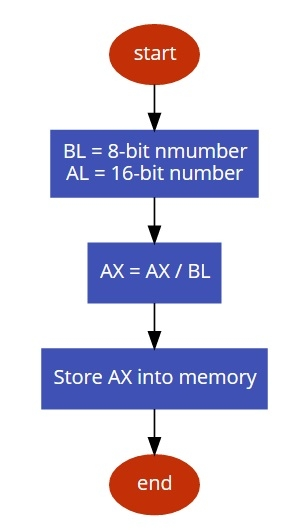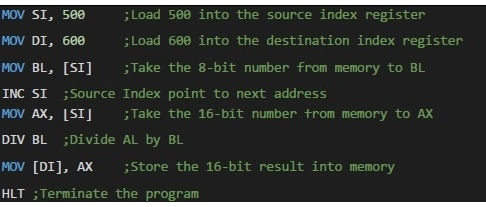# 8086 program to divide a 16 bit number by an 8 bit number

MicroprocessorMicrocontroller8086

#### Microprocessor 8085

50 Lectures 6.5 hours

In this program we will see how to divide 16-bit number by an 8-bit number.

## Problem Statement

Write 8086 Assembly language program to divide 16-bit number stored in memory location offset 501. Divide it with 8-bit number stored in 500H. Also store result at memory offset 600.

## Discussiont

8086 has DIV instruction to perform division. Take the 8-bit number into BL, and 16-bit number into AX. Now divide AX by BL. The result will be stored at AX.

We are taking two numbers 24CF / 2D = D1

Data

500
2D
501
CF
502
24

## Flow Diagram## Program